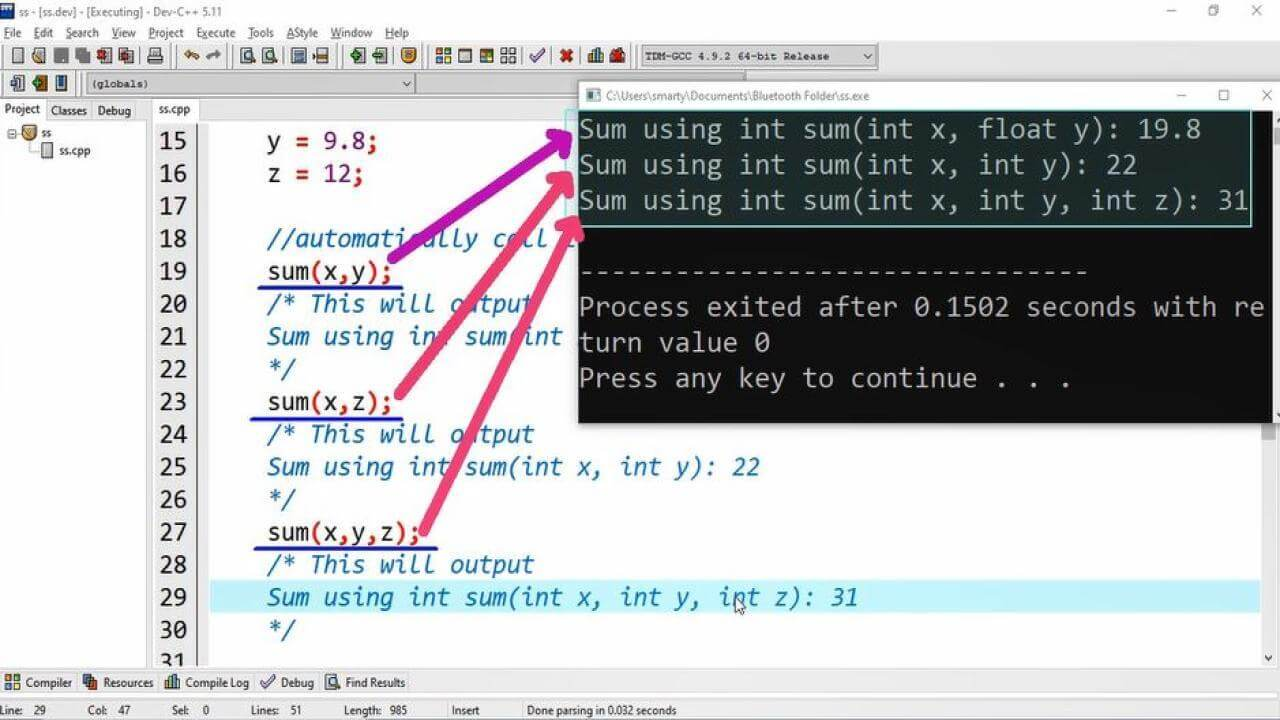## Source Code:

#include <iostream>
using namespace std;

int sum(int, int); // function declaration
int sum(int, int, int);
float sum(int, float);

int main(int argc, char** argv) {

int x;
float y;
int z;
x = 10;
y = 9.8;
z = 12;

//automatically call to appropriate function
sum(x,y);
/* This will output
Sum using int sum(int x, float y): 19.8
*/

sum(x,z);
/* This will output
Sum using int sum(int x, int y): 22
*/

sum(x,y,z);
/* This will output
Sum using int sum(int x, int y, int z): 31
*/

return 0;
}

int sum(int x, int y) { // function Header
cout<<"Sum using int sum(int x, int y): ";
cout<<x+y<<endl;

}
float sum(int x, float y) { // function Header
cout<<"Sum using int sum(int x, float y): ";
cout<<x+y<<endl;

}
int sum(int x, int y, int z) { // function Header
cout<<"Sum using int sum(int x, int y, int z): ";
cout<<x+y+z<<endl;

## } Output:## Working:

In this program example we declare three functions having same name but different no of parameters and parameters names. In C++ function overloading can also be achieved by different return types.

The detail of these function is as provided:

 Sr. Return Type Function Name No of Parameters Parameters Types 1 int sum 2 int, int 2 int sum 3 int, int, int 3 float sum 2 int, float

After functions declaration we declare three variables two of int type and one of float data type. Compile will automatically bind appropriate function depending on no of parameters provided at the time of function call during compilation process. This is also called early binding

In main function we call sum function provided on different no of parameters. In this program we define function after main() function.